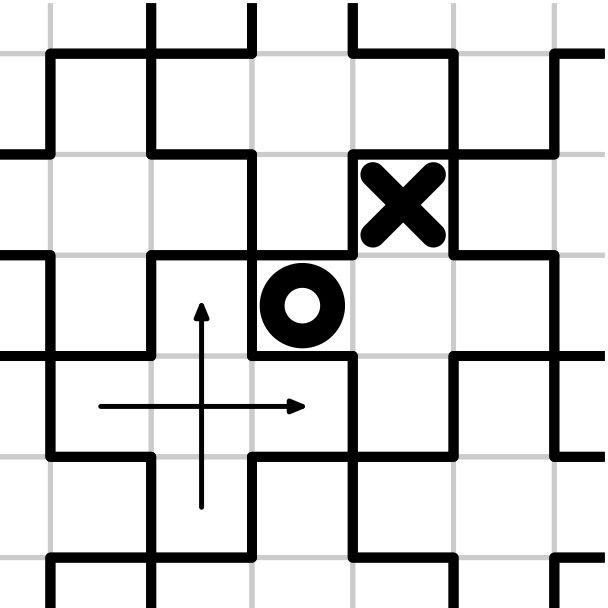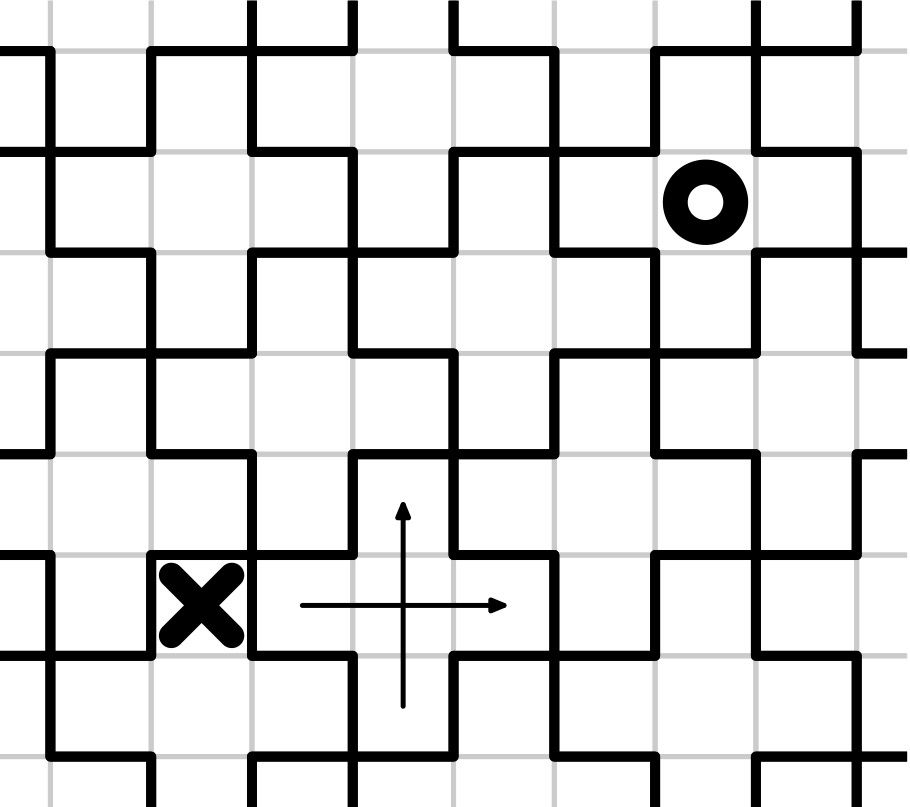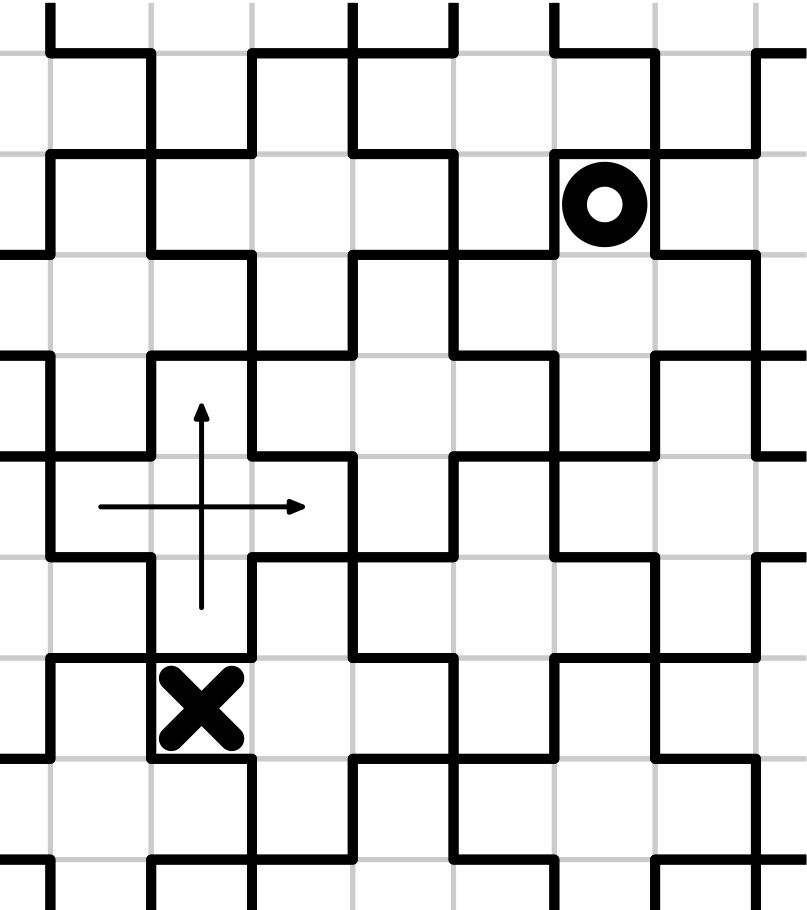시간 제한메모리 제한제출정답맞힌 사람정답 비율
2 초 (추가 시간 없음) 512 MB111100.000%

## 문제

Consider a plane partitioned into squares with side $1$. Let us choose a square and draw coordinate axes from its center parallel to its sides.

Next, let us draw a cross consisting of the central square and its four neighbors: the squares which share a side with it. Then pick the square centered at point $(2, 1)$ and draw another cross consisting of this square and its four neighbors. Tile the whole plane with such crosses: their centers will be in points with coordinates $(2 i + j, i - 2 j)$ for all possible integer $i$ and $j$. The tiling is shown alongside the examples.

Emilia stands at the center of some square on the plane. In one step, she can move from a square to one of its neighbors. If a step brings her to a different cross of the tiling, she has to pay one coin for this step. Steps such that Emilia remains in the same cross are free.

Let the distance in crosses between two squares $A$ and $B$ be the minimum possible number of coins that Emilia has to pay in order to get from $A$ to $B$. You are given the coordinates of two points on the plane: center of the initial square and center of the target square. Find the distance in crosses between them.

## 입력

The first line contains two integers $x_1$ and $y_1$, the coordinates of the initial square. The second line contains two integers $x_2$ and $y_2$, the coordinates of the destination square. All given coordinates do not exceed $10^{9}$ by absolute value.

## 출력

Print one integer: the distance in crosses from the initial square to the destination square.

## 예제 입력 1

1 1
2 2


## 예제 출력 1

0## 예제 입력 2

3 4
-2 0


## 예제 출력 2

4## 예제 입력 3

4 3
0 -2


## 예제 출력 3

3